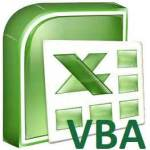# Access Excel remove text in bracketsThis Access Excel VBA tutorial explains how to remove text in brackets using custom Function. You may also want to read: Change text color in brackets Extract text in brackets Access Excel remove text in brackets I was inspired to create this post because the HR system I am using (Workday) is very annoying, some data fields are actually a combination of two different fields, the additional data field is displayed in brackets in the prefix. For example,  an employee has a English name ...
More

# Access Excel INT Function to get the integer of numberThis Access / Excel tutorial explains how to use INT Function to get the integer part of a number. Access Excel INT Function to get the integer of number When you have a number with decimal places, you can separate the number into integer part using INT Function, and then work around to get the decimal part. INT Function can be used in Access and Excel, and also VBA. Syntax of INT Function INT(number) INT Function only contains one argument, which is the number from which you want to get th...
More

# Excel VBA NPV Function to calculate Net Present ValueThis Excel VBA tutorial explains how to calculate net present value using Excel VBA NPV Function. You may also want to read: Excel worksheet NPV Function Net Present Value Net Present Value is to calculate the present value of future cash flow. For example, your client will give you \$10000 when the project completes after 1 year, assume there will be inflation, the future \$10000 will be less than the present \$10000. Assume the inflation is 4%, the present value of \$10000 is 10000/(1+4%) ...
MoreMore

# Excel VBA DIR FunctionThis Excel tutorial explains how to use Excel VBA DIR Function and loop through workbooks in a folder. You may also want to read: Excel loop workbooks in folders and subfolders with FileSystemObject Excel VBA DIR Function DIR Function returns the first file name that matches the criteria in Function argument (pathname and attributes). DIR Function is usually used with loop in order to manipulate multiple files of specific name. Syntax of DIR Function Dir(pathname, [attributes]) ...
More

# Excel VBA Inputbox and Application.InputboxThis Excel tutorial explains difference between VBA Inputbox and  Application.Inputbox and how to use them. You may also want to read: Excel VBA Msgbox and handling of return value Excel VBA use Inputbox to select options Excel VBA Inputbox and Application.Inputbox Inputbox is a pop up dialog box that displays a message and provides a text box for users to input free text. It is very similar to Msgbox except that Msgbox does not have a text box for user input. There are two kinds o...
More

# Excel VBA Rnd Function to generate random numberThis Excel tutorial explains how to use Excel VBA Rnd Function to generate random number and create custom Function for random decimal number. You may also want to read: Excel RAND RANDBETWEEN Function to generate random number Access Excel Generate random HKID Hong Kong ID card number Assign unique random number using Excel Excel VBA Rnd Function to generate random number Rnd Function is for use in Access, Access VBA, Excel VBA, it is used to generate random number larger than 0 and s...
More

# VBA Excel Split Function to delimit textWhat does VBA Excel Split Function do? VBA Excel Split Function separates a text by delimiter into an array, for example, you can split a text a;b;c;d into array {a,b,c,d}, afterwards you can get the desired array item (starting from 0). Split Function is similar to the action of "Text to Columns". Syntax of VBA Excel Split Function Split(text,[delimiter],[limit],[compare]) text text to be delimited delimiter Optional. String character used to identify substring limits. If omit...
More

# Access Excel VBA Chr Function to convert ASCII value to characterThis Access Excel VBA tutorial explains how to use Chr Function to convert ASCII value to character, and convert from character to ASCII value with ASC Function What is Access Excel VBA Chr Function? Access Excel VBA Chr Function converts ASCII value to a character. ASCII (American Standard Code for Information Interchange) uses 8-bit code units, an old encoding system which stores mainly numbers, lowercase letters a to z, uppercase letters A to Z, basic punctuation symbols, control codes. M...
More

# Excel VBA Msgbox and handling of return valueThis Excel tutorial explains how to use Excel VBA Msgbox with carriage return such as vbCr, vbNewLine, and handling of return value such as vbOK, vbCancel, vbRetry You may also want to read: Excel VBA Inputbox and Application.Inputbox What is Excel VBA MsgBox? Excel VBA MsgBox is a prompt box that is used to prompt user for important information, usually provide user with OK and Cancel buttons. Note that there is another prompt box called InputBox which receives text input from user but ...
More